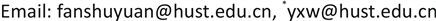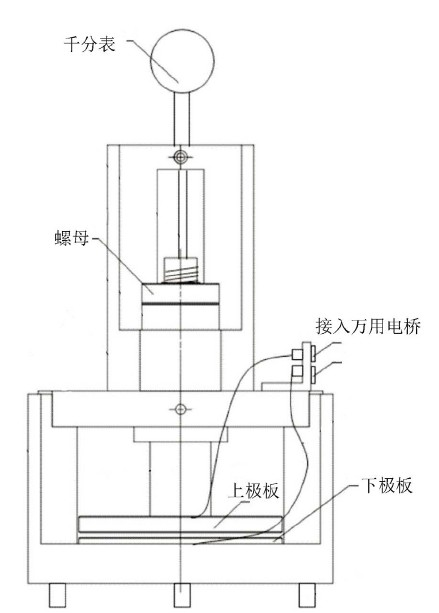﻿ 固体介质相对介电常数测量实验装置的改进 Improvement of Experimental Device for Measuring Relative Dielectric Constant of Solid Medium

Instrumentation and Equipments
Vol.06 No.03(2018), Article ID:26583,5 pages
10.12677/IaE.2018.63010

Improvement of Experimental Device for Measuring Relative Dielectric Constant of Solid Medium

Shuyuan Fan, Xiaowu Yu*

School of Materials Science and Engineering, Huazhong University of Science and Technology, Wuhan HubeiReceived: Aug. 5th, 2018; accepted: Aug. 20th, 2018; published: Aug. 27th, 2018ABSTRACT

Some problems are analyzed existing in the teaching instrument for the experiment of measuring the relative dielectric constant of solid media. To solve these problems, the experimental measuring device is redesigned. In the new device, the upper plate of the parallel plate capacitor is not driven by the spiral micrometer to move up and down, but is driven by the rotation of a stop nut. The upper plate does not rotate when it is moving up and down. The size of the plate is no longer affected by the design scheme and can be easily made larger. A micrometer gauge is used for the real-time measurement of the moving distance of the upper plate. The improved experimental device solves a series of problems in the original device and improves the accuracy of the experiment.

Keywords:Dielectric Constant, Parallel Plate Capacitor, Experiment Teaching1. 引言

2. 现有固体介质材料相对介电常数测量装置存在的问题

2.1. 固体介质材料相对介电常数测量方法

${\epsilon }_{r}=\frac{C}{{C}_{0}}$ (1)

${C}_{1}={C}_{0}+{C}_{边缘}+{C}_{分布}$ (2)

${C}_{2}={C}_{串}+{C}_{边缘}+{C}_{分布}$ (3)Figure 1. The principle diagram of the relative dielectric constant solid dielectric material experiment

${C}_{串}$ 为空气和被测样品串联后理论上两极板间的电容， ${C}_{边缘}$ 为电介质样品以外的边缘电极间的电容量， ${C}_{分布}$ 为测量系统所含有的分布电容    。

${C}_{串}={C}_{2}-{C}_{1}+{C}_{0}={C}_{2}-{C}_{1}+\frac{{\epsilon }_{0}S}{D}$ (4)

${C}_{串}=\frac{\frac{{\epsilon }_{0}S}{D-t}\frac{{\epsilon }_{r}{\epsilon }_{0}S}{t}}{\frac{{\epsilon }_{0}S}{D-t}+\frac{{\epsilon }_{r}{\epsilon }_{0}S}{t}}=\frac{{\epsilon }_{r}{\epsilon }_{0}S}{t+{\epsilon }_{r}\left(D-t\right)}$ (5)

${\epsilon }_{r}=\frac{{C}_{串}t}{{\epsilon }_{0}S-{C}_{串}\left(D-t\right)}$ (6)

2.2. 现有的实验装置中存在的一些问题

1) 将上极板固定在一个螺旋测微仪上，通过旋转螺旋测微仪，来控制上极板与下极板的间距，这种结构决定了电解板不能做得太大，一般直径为d = 40 mm左右，在该方法中，理论上要求电极板直径d远大于电极板间距D   ，在实验中，为了保证实验精度，要求 $D\le 0.05d$

2) 在该结构中，样品的厚度只能控制在1~2 mm，要求厚度均匀、表明平整。但是，直径为40 mm、厚度为1~2 mm的样品要达到该要求，加工难度大，容易变形，而且样品厚度测量的相对误差比较大  。所以实验中，只能够用厂家提供的特制样品。

3) 由于两极板很做成绝对相互平行，通过旋转螺旋测微仪，来控制上极板上下移动位置，极板会发生转动，那么整过实验过程中，仪器的系统误差是不断改变的。

3. 固体介质材料相对介电常数测量装置的改进

1) 转动螺母，使上极板与下极板的间距为零，按下千分表的归零键。

2) 转动螺母，使上极板上升略大于被测材料厚度的高度，平行放入被测材料，转动螺母使上极板下降并压紧被测材料，读取此时的千分表示数并记录。重复该步骤5次，每次使上极板上升后都将被测介质以中心线为轴转动一定的角度再压紧，分别记录5次的千分表示数，平均值为被测样品的厚度t。

3) 转动螺母使上极板略微上升，取出被测材料，待万用电桥示数稳定后记录读数 ${C}_{1}$ ，同时记下此时的千分表读数D。保持螺母不动，重新将被测材料平行放入上极板与下极板之间，待万用电桥示数稳定后记录读数 ${C}_{2}$

4) 重复第3步5次，分别测出上下极板不同D状态下的 ${C}_{1}$${C}_{2}$ 值。

4. 实验结果分析Figure 2. The device of solid material relative dielectric constant measurementTable 1. The measurements of the relative dielectric constant of organic glassTable 2. The data processing results of the organic glass relative dielectric constant measurement data

5. 结论

1) 保证了系统误差恒定，改进后的装置因上极板上下移动时不再发生转动；

2) 保证了电极板直径远大于电极板间距的实验模型，极板的大小不再受到结构的限制，做得更大，本装置中极板的直径为100.00 mm，样品厚度可在2~4 mm内，都可以有比较好的精度；

3) 提高了样品厚度的相对测量精度，样品厚度在2~4 mm间，相对测量厚度精度提高，且样品的平行度更容易保证。

Improvement of Experimental Device for Measuring Relative Dielectric Constant of Solid Medium[J]. 仪器与设备, 2018, 06(03): 69-73. https://doi.org/10.12677/IaE.2018.63010

1. 1. 任忠明, 等, 主编. 大学物理实验第二册[M]. 北京: 科学出版社, 2008: 55-57.

2. 2. 叶伟国. 有电介质时平行板电容器电容计算问题的讨论[J]. 物理与工程, 2008, 18(3): 63-64.

3. 3. 浦天舒, 杨旭方, 郭程, 等. 电容器边缘效应对介电常数测量的影响及修正[J]. 大学物理实验, 2013, 26(3): 46-47.

4. 4. 朱兆青. 利用单片机测量介电常数[J]. 实验室研究与探索, 2005, 24(6): 48-50.

5. 5. 何捷. 空气介电常数ε0的测定[J]. 南京师大学报(自然科学版), 1986, 1(1): 86-88.

6. 6. 唐海燕, 等, 主编. 工科物理教程上册[M]. 北京: 国防工业出版社, 2007: 240-249.

NOTES

*通讯作者。